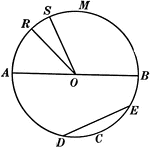### Chords, Diameters, and Radii of a Circle

Illustration of a circle with diameter AB. Radii are RO, SO, AO, and BO. ED is a chord.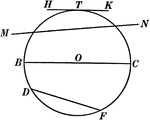### Chords, Secants, Diameters, and Tangents of a Circle

Illustration of a circle with diameter BC, chord DF, secant MN, and tangent HK.### Circle With Diameter and Chord

Illustration of a circle with diameter and chord drawn. A diameter perpendicular to a chord bisects…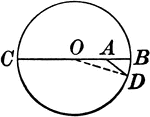### Circle With Diameter, Radius, Segment

Illustration showing a circle with a diameter, radius, and segment drawn.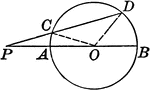### Circle With Diameter, Radius, Segment, Line

Illustration showing a circle with a diameter, radius, lines, triangle, and segment drawn.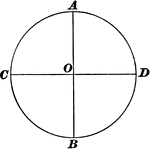### Diameters of a Circle

Illustration of a circle with two intersecting diameters.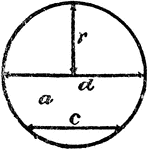### Radius, Diameter, and Chord In A Circle

An illustration showing a circle with radius r, diameter d, and chord c.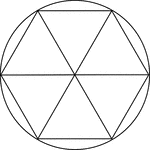### Regular Hexagon Inscribed In A Circle

Illustration of a regular hexagon inscribed in a circle. This can also be described as a circle circumscribed…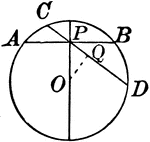### Circle With Various Chords

Illustration showing a circle with various chords drawn.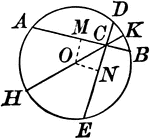### Circle With Various Chords

Illustration showing a circle with various chords drawn.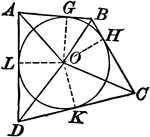### Circle With Various Chords And Tangents

Illustration showing a circle with various chords and tangents drawn.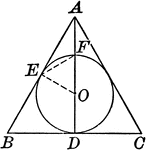### Circle With Various Chords And Tangents

Illustration showing a circle with various chords and tangents drawn.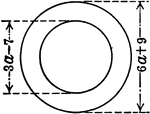### Ring Made of Circles With Diameters 3a-7 and 6a+9

An illustration of a ring (circle within circle), with diameters of 3a-7 and 6a+9. Illustration could…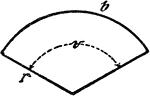### Circle Sector

An illustration showing a circle sector with radius r, center/central angle v, and length of circle…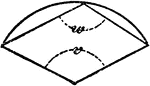### Circle Sector

An illustration showing a circle sector with center/central angle v and polygon angle w.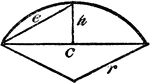### Circle Sector

An illustration showing a circle sector with height of segment h and radius r.